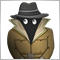# OrderTotal count is wrong in strategy tester all the time?10

In my strategy I have the following code:

```void OnTick() {

// Stuff

if(signal() && OrdersTotal() < 1) {

OrderSend(Symbol(),
calculatedLotSize,
calculatedSlippageRisk,
Bid + takeProfit * Point,
"MY ORDER",
magicNum,
Green);
}

}```

My code generates a lot of signals and therefore a lot of trades. Due to the type of signal it can get "hung up" if the chart bounces off of the point of the signal a few times. I've been reading the forums and come across a few topics (including counting with OrderSelect and such) and these have not helped at all.

Placing tons of orders is undesirable and I'd like to limit my total outstanding orders to 1. So I wrote some code to count orders:

``` int OrderCount() {
int count = 0;
for(int i = OrdersTotal() - 1; i >= 0; i--){
if(OrderMagicNumber() == magicNum
&& OrderSymbol() == Symbol()
&& (OrderType() == OP_BUY || OrderType() == OP_SELL)) {
count++;
}
} else {
Print("Error Selecting Order - #", GetLastError());
}
}

return count;
}

```

This should count my orders. Modifying the above OnTick code the condition:

```if(signal() && OrderCount() < 1)

```

Should only work if there are no outstanding (pending or otherwise) orders. However, this doesn't work at all. In backtesting in the strategy tester it generates thousands of signals over a period of time. Putting counters in the code demonstrates OrderCount() oscillates between 0, and 1. This leaves me to think of a few things:

1. Somehow OrdersTotal() doesn't work in the strategy tester
2. Somehow my OrderCount() function only counts successfully executed orders (which is why it floats between 0, and 1)
3. Pending orders that haven't been filled yet aren't being counted.

Is there a better way to approach this? I've tried browsing the MQL4 forums for basically hours and I can't find a solution to this.7864

```void OnTick()
{
ticket = 0;

if(signal())
if(OrderCount() == 0)
ticket = OrderSend(Symbol(),
calculatedLotSize,
calculatedSlippageRisk,
(stopLoss == 0   ? 0 : stopLevel > stopLoss   ? (Ask - stopLevel*Point) : (Ask - stopLoss*Point)),
(takeProfit == 0 ? 0 : stopLevel > takeProfit ? (Ask + stopLevel*Point) : (Ask + takeProfit*Point)),
"MY ORDER",
magicNum,
Green);

}

int OrderCount()
{
int count = 0;
for(int i = OrdersTotal() - 1; i >= 0; i--){
if(OrderMagicNumber() != magicNum)              continue;
if(OrderSymbol() != Symbol())                   continue;
if(OrderType() == OP_BUY || OrderType() == OP_SELL)
count++;
}
return count;
}```20210

1. ```                  Ask - stopLoss * Point,
Bid + takeProfit * Point,```
• Your sell order's TP/SL will be triggered when the Ask reaches it. To trigger at a specific Bid price, add the average spread.
MODE_SPREAD (Paul) - MQL4 and MetaTrader 4 - MQL4 programming forum - Page 3
• The charts show Bid prices only. Turn on the Ask line to see how big the spread is (Tools -> Options {control-O} -> charts -> Show ask line.)

2. _one: This should count my orders. Modifying the above OnTick code the condition:
`if(signal() && OrderCount() < 1)`

Should only work if there are no outstanding (pending or otherwise) orders. However, this doesn't work at all. In backtesting in the strategy tester it generates thousands of signals over a period of time. Putting counters in the code demonstrates OrderCount() oscillates between 0, and 1. This leaves me to think of a few things:

1. Somehow OrdersTotal() doesn't work in the strategy tester
2. Somehow my OrderCount() function only counts successfully executed orders (which is why it floats between 0, and 1)
3. Pending orders that haven't been filled yet aren't being counted.
3. Your code is working exactly as coded. PICNIC
1. OrdersTotal works just fine. Do you think you are the first person in twenty years to try it in the tester?
2. `if(OrderType() == OP_BUY || OrderType() == OP_SELL) count++;`
What orders do you think your code is counting?
3. What order type do you think pending orders are? Perhaps you should read the manual.
Order Properties - Trade Constants - Standard Constants, Enumerations and Structures - MQL4 Reference10

whroeder1:
• Your sell order's TP/SL will be triggered when the Ask reaches it. To trigger at a specific Bid price, add the average spread.
MODE_SPREAD (Paul) - MQL4 and MetaTrader 4 - MQL4 programming forum - Page 3
• The charts show Bid prices only. Turn on the Ask line to see how big the spread is (Tools -> Options {control-O} -> charts -> Show ask line.)

2. Your code is working exactly as coded. PICNIC
1. OrdersTotal works just fine. Do you think you are the first person in twenty years to try it in the tester?
2. What orders do you think your code is counting?
3. What order type do you think pending orders are? Perhaps you should read the manual.
Order Properties - Trade Constants - Standard Constants, Enumerations and Structures - MQL4 Reference

You sure do have a way with words don't you.

For (2), the problem was there were so many ticks I was missing counts in the logs. My TP/SL were getting hit really quickly, and widening my risk/reward helped reduce my trades. I had misattributed it to my OrdersTotal code. Once I had analyzed the entries and exits. Indeed this is PEBKAC, I'm not used to this strategy tester if it wasn't obvious.

For the SL - thanks for catching that. I'm not sure why I put that there. Of course it would be bid.